+关注继续查看

# 一、函数

#include "stdio.h"
void main()
{
//write once only once 只写一次
int i,s=0;

for(i=1;i<=100;i++)
{
s+=i;
}
printf("%d \n",s);

s=0;
for(i=1;i<=80;i++)
{
s+=i;
}
printf("%d \n",s);

s=0;
for(i=1;i<=555;i++)
{
s+=i;
}
printf("%d \n",s);
}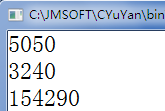#include "stdio.h"

/*

int 表示函数的返回值（return），没返回值void
sum 表示函数的名称，符合变量命名
(int n) 参数列表，可以有0-n个
*/
int sum(int n)
{
return n+1;
}

void main()
{
int x=sum(100);
x=sum(x);
printf("%d \n",x);  //函数调用
printf("%d \n",sum(9));
}

102

10

#include "stdio.h"

/*

int 表示函数的返回值（return），没返回值void
sum 表示函数的名称，符合变量命名
(int n) 参数列表，可以有0-n个
*/
int sum(int n)
{
int i,s=0;
for(i=1;i<=n;i++)
{
s+=i;
}
return s;
}

void main()
{
printf("%d \n",sum(100));
printf("%d \n",sum(80));
printf("%d \n",sum(555));
}## 1.2.1、函数定义语法

函数类型  函数名(类型 参数名，类型 参数名...)
{
函数体;
}

void show(char c)
{
printf("%c\n",c);
}

## 1.2.2、函数调用

void main()
{
show('a');
show('b');
show('c');
}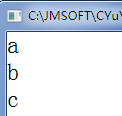write once only once

#include "stdio.h"

//定义函数
//int 表示函数的返回值,无返回值void，return返回值
//sum 表示函数名称，与变量命名规则相同
//int n表示参数，可以有0-n个，形参
int sum(int n)
{
int s=0,i;
for(i=1;i<=n;i++)
{
s+=i;
}
return s;
}

void p(int n){
printf("%d \t",n);
}

void l()
{
printf("\n");
}

void main()
{
p(sum(100));
l();
p(sum(300));
l();
p(sum(1000));
}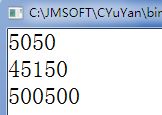## 1.3、递归

int f(int n)
{
if(n==1) return 1;
return f(n-1)+n;
}

void main()
{
printf("%d",f(100));
}

5050

f(n)=f(n-1)+n;

//1 1 2 3 5 8 13 21 34
/* function
f(n)
f(5)=5
f(6)=8
f(7)=13
f(n)=f(n-1)+f(n-2)
f(7)=f(6)+f(5)
13=8+5
*/
int f(int n)  //n第几位
{
if(n==1 || n==2) return 1;
return f(n-1)+f(n-2);
}

void main()
{
printf("%d",f(45));
}

# 二、指针

## 2.1、指针的概念

#include "stdio.h"
void main()
{
int n=100;
int *p=&n;

printf("%d \n",p);  //输入逻辑地址
printf("%p \n",p);  //%p 是以16进制的形式输出内存地址
}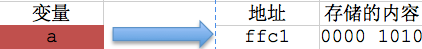## 2.2、指针的定义

int *p;
char *q;

"*"是一个说明符，用来说明这个变量是个指针变量，是不能省略的，但它不属于变量名的一部分

## 2.3、指针的初始化

// 定义int类型的变量a
int a = 10;

// 定义一个指针变量p
int *p;

// 将变量a的地址赋值给指针变量p，所以指针变量p指向变量a
p = &a;

// 定义int类型的变量a
int a = 10;

// 定义一个指针变量p
// 并将变量a的地址赋值给指针变量p，所以指针变量p指向变量a
int *p = &a;

## 2.4、指针运算符

char a = 10;
printf("修改前，a的值：%d\n", a);

// 指针变量p指向变量a
char *p = &a;

// 通过指针变量p间接修改变量a的值
*p = 9;

printf("修改后，a的值：%d", a);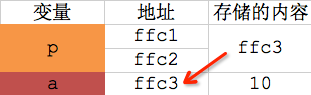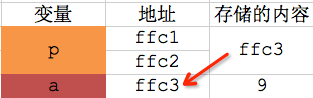char a = 10;

char *p;
p = &a;

char value = *p;
printf("取出a的值：%d", value);

/* Note:Your choice is C IDE */
#include "stdio.h"
void main()
{
int n=99;
int *p=&n;  //定义指针p，可以指向int类型变量的地址
//&n是取出n的地址

printf("%d\n",p);
printf("%p\n",p);

printf("%d \n",*p);  //取出地址对应的值

*p=55;  //将p指向的地址对应的值修改为55

printf("%d\n",n);

printf("%d \n",*p);  //取出地址对应的值

}

## 2.5、指针与数组

int array={0,1,2,3,4,5,6,7,8,9},value;

array就是数组的地址。

value=array;//也可写成：value=*array;
value=array;//也可写成：value=*(array+3);
value=array;//也可写成：value=*(array+4);

int array;
int (*ptr);   //指向地址的地址
ptr=&array;

，我们用整个数组的首地址来初始化它。在语句ptr=&array中，array代表数组本

int arrays={1,2,3};
int (*ptr);
ptr=&arrays;

printf("%d\n",**ptr+3);  //指向地址的地址

# 三、宏

C语言中提供的宏定义命令,其主要目的是为程序员在编程时提供一定的方便,并能在一定程度上提高程序的运行效率。

## 3.1、简单宏定义

[#define指令（简单的宏）]　　#define 标识符替换列表

#define STE_LEN 80
#define TRUE 1
#define FALSE 0
#define PI 3.14159

## 3.2、带参数的宏

[#define指令—带参数的宏]　　#define 标识符（x1, x2,…,xn）替换列表

#define MAX(x,y)    ((x)>(y) ? (x) :(y))
#define IS_EVEN(n)   ((n)%2==0)  

i = MAX(j+k, m-n);
if (IS_EVEN(i)) i++;  

i = ((j+k)>(m-n)?(j+k):(m-n));
if (((i)%2==0)) i++;  

200

#define PRINT_INT(x)    printf("%d\n", x)

## 3.3、C语言中常用的宏

01: 防止一个头文件被重复包含
#ifndef COMDEF_H
#define COMDEF_H
//头文件内容
#endif

02: 重新定义一些类型

typedef unsigned char boolean; /* Boolean value type. */
typedef unsigned long int uint32; /* Unsigned 32 bit value */
typedef unsigned short uint16; /* Unsigned 16 bit value */
typedef unsigned char uint8; /* Unsigned 8 bit value */
typedef signed long int int32; /* Signed 32 bit value */
typedef signed short int16; /* Signed 16 bit value */
typedef signed char int8; /* Signed 8 bit value */

//下面的不建议使用
typedef unsigned char byte; /* Unsigned 8 bit value type. */
typedef unsigned short word; /* Unsinged 16 bit value type. */
typedef unsigned long dword; /* Unsigned 32 bit value type. */
typedef unsigned char uint1; /* Unsigned 8 bit value type. */
typedef unsigned short uint2; /* Unsigned 16 bit value type. */
typedef unsigned long uint4; /* Unsigned 32 bit value type. */
typedef signed char int1; /* Signed 8 bit value type. */
typedef signed short int2; /* Signed 16 bit value type. */
typedef long int int4; /* Signed 32 bit value type. */
typedef signed long sint31; /* Signed 32 bit value */
typedef signed short sint15; /* Signed 16 bit value */
typedef signed char sint7; /* Signed 8 bit value */

03: 得到指定地址上的一个字节或字

#define MEM_B(x) (*((byte *)(x)))
#define MEM_W(x) (*((word *)(x)))

04: 求最大值和最小值

#define MAX(x,y) (((x)>(y)) ? (x) : (y))
#define MIN(x,y) (((x) < (y)) ? (x) : (y))
05: 得到一个field在结构体(struct)中的偏移量

#define FPOS(type,field) ((dword)&((type *)0)->field)

06: 得到一个结构体中field所占用的字节数
#define FSIZ(type,field) sizeof(((type *)0)->field)
07: 按照LSB格式把两个字节转化为一个Word

#define FLIPW(ray) ((((word)(ray)) * 256) + (ray))

08: 按照LSB格式把一个Word转化为两个字节
#define FLOPW(ray,val) (ray) = ((val)/256); (ray) = ((val) & 0xFF)
09: 得到一个变量的地址（word宽度）

#define B_PTR(var) ((byte *) (void *) &(var))
#define W_PTR(var) ((word *) (void *) &(var))

10: 得到一个字的高位和低位字节
#define WORD_LO(xxx) ((byte) ((word)(xxx) & 255))
#define WORD_HI(xxx) ((byte) ((word)(xxx) >> 8))

11: 返回一个比X大的最接近的8的倍数
#define RND8(x) ((((x) + 7)/8) * 8
12: 将一个字母转换为大写

#define UPCASE(c) (((c)>='a' && (c) <= 'z') ? ((c) – 0×20) : (c))
13: 判断字符是不是10进值的数字

#define DECCHK(c) ((c)>='0' && (c)<='9')
14: 判断字符是不是16进值的数字

#define HEXCHK(c) (((c) >= '0' && (c)<='9') ((c)>='A' && (c)<= 'F') \
((c)>='a' && (c)<='f'))

15: 防止溢出的一个方法
#define INC_SAT(val) (val=((val)+1>(val)) ? (val)+1 : (val))

16: 返回数组元素的个数
#define ARR_SIZE(a) (sizeof((a))/sizeof((a)))

17: 返回一个无符号数n尾的值MOD_BY_POWER_OF_TWO(X,n)=X%(2^n)
#define MOD_BY_POWER_OF_TWO( val, mod_by ) ((dword)(val) & (dword)((mod_by)-1))

18: 对于IO空间映射在存储空间的结构,输入输出处理
#define inp(port) (*((volatile byte *)(port)))
#define inpw(port) (*((volatile word *)(port)))
#define inpdw(port) (*((volatile dword *)(port)))
#define outp(port,val) (*((volatile byte *)(port))=((byte)(val)))
#define outpw(port, val) (*((volatile word *)(port))=((word)(val)))
#define outpdw(port, val) (*((volatile dword *)(port))=((dword)(val)))

19: 使用一些宏跟踪调试
ANSI标准说明了五个预定义的宏名。它们是：
__LINE__
__FILE__
__DATE__
__TIME__
__STDC__

C++中还定义了 __cplusplus

__LINE__ 及 __FILE__ 宏指示，#line指令可以改变它的值，简单的讲，编译时，它们包含程序的当前行数和文件名。
__DATE__ 宏指令含有形式为月/日/年的串,表示源文件被翻译到代码时的日期。
__TIME__ 宏指令包含程序编译的时间。时间用字符串表示，其形式为： 分：秒
__STDC__ 宏指令的意义是编译时定义的。一般来讲，如果__STDC__已经定义，编译器将仅接受不包含任何非标准扩展的标准C/C++代码。如果实现是标准的,则宏__STDC__含有十进制常量1。如果它含有任何其它数,则实现是非标准的。
__cplusplus 与标准c++一致的编译器把它定义为一个包含至少6为的数值。与标准c++不一致的编译器将使用具有5位或更少的数值。

#ifdef _DEBUG
#define DEBUGMSG(msg,date) printf(msg);printf(“%d%d%d”,date,_LINE_,_FILE_)
#else
#define DEBUGMSG(msg,date)
#endif
20： 宏定义防止错误使用小括号包含。

else
do_somethong_else();
//宏展开以后变成这样:
{memcpy(bufp,addr,nr); bufp += nr;};
else
do_something_else();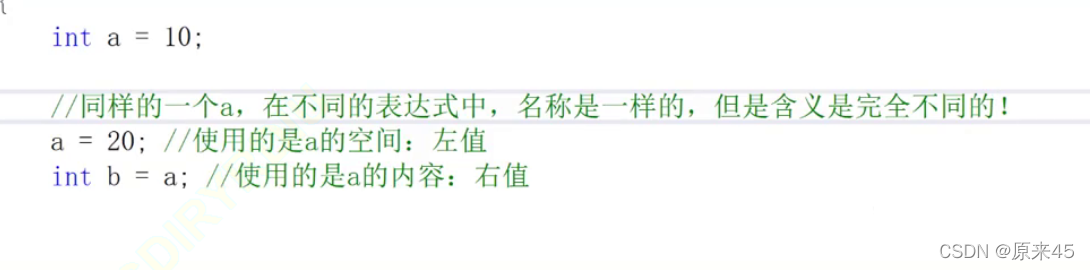《C语言深度剖析》第四章 指针和数组 p1 C语言从入门到入土（进阶篇）（一）

41 0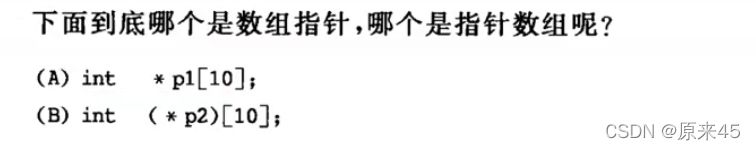《C语言深度剖析》第四章 指针和数组 p4 C语言从入门到入土（进阶篇）

38 0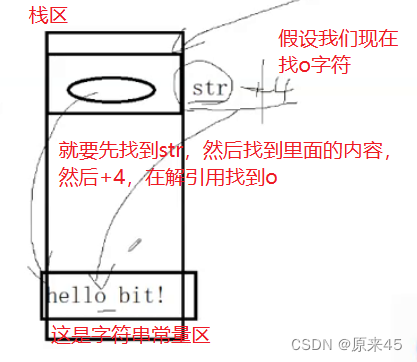《C语言深度剖析》第四章 指针和数组 p3 C语言从入门到入土（进阶篇）

16 0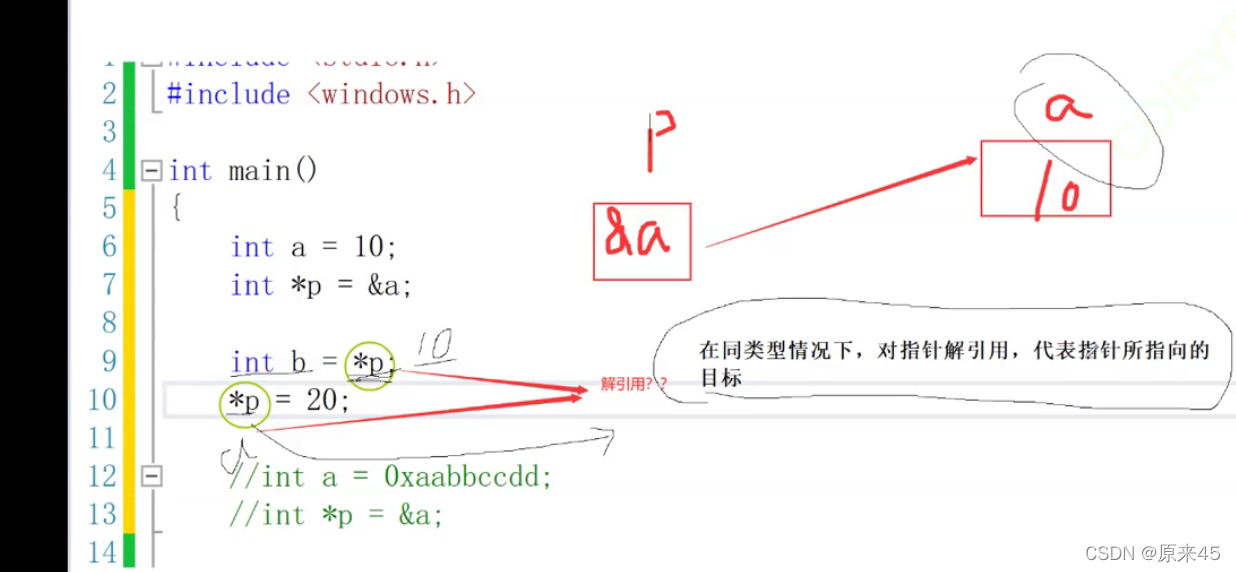《C语言深度剖析》第四章 指针和数组 p1 C语言从入门到入土（进阶篇）（二）

48 0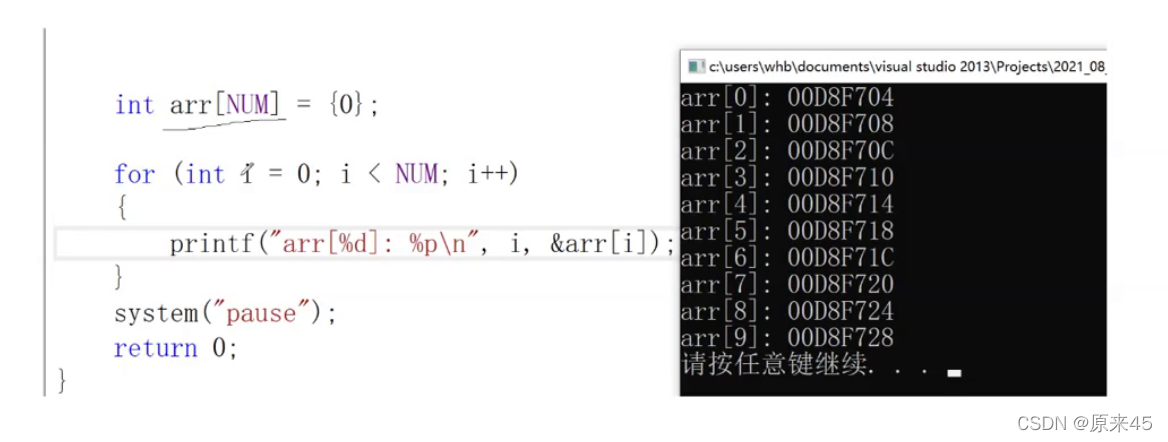《C语言深度剖析》第四章 指针和数组 p2 C语言从入门到入土（进阶篇）

24 0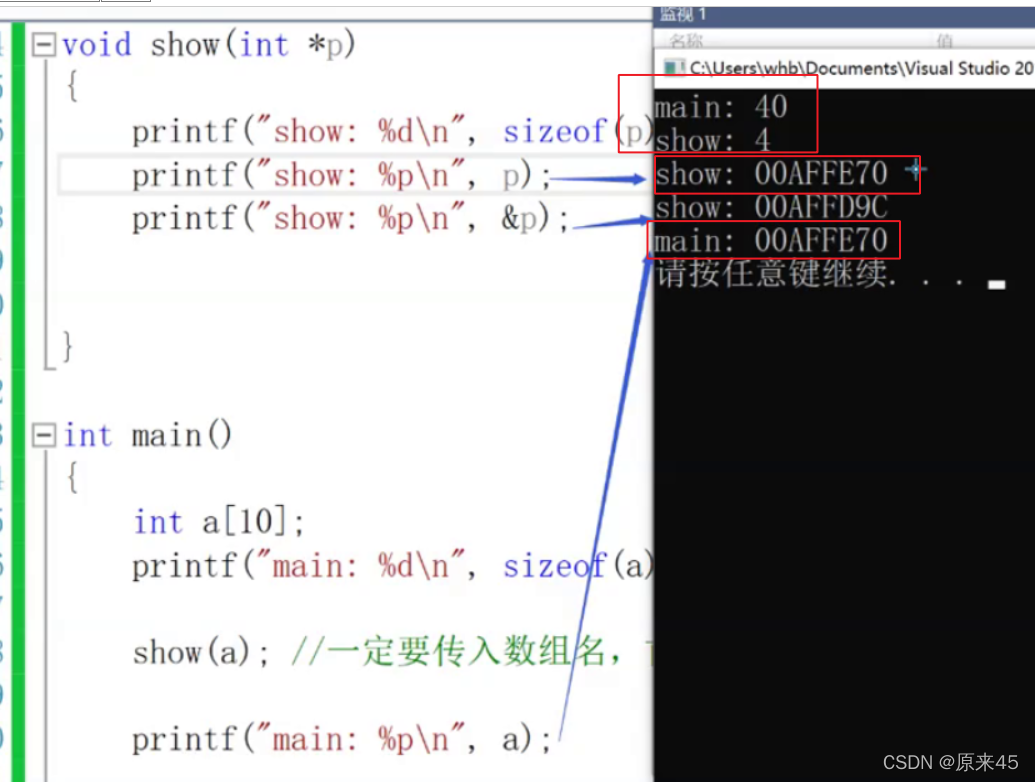《C语言深度剖析》第四章 指针和数组 p5(完结)（ C语言从入门到入土（进阶篇）（一）

27 0「C语言回顾之旅」第一篇：指针详解
964 0C语言指针强制类型转换
1571 0「C语言回顾之旅」第二篇：指针详解进阶
967 0C语言的指针、数据、结构体关系总结
1120 0

35

0